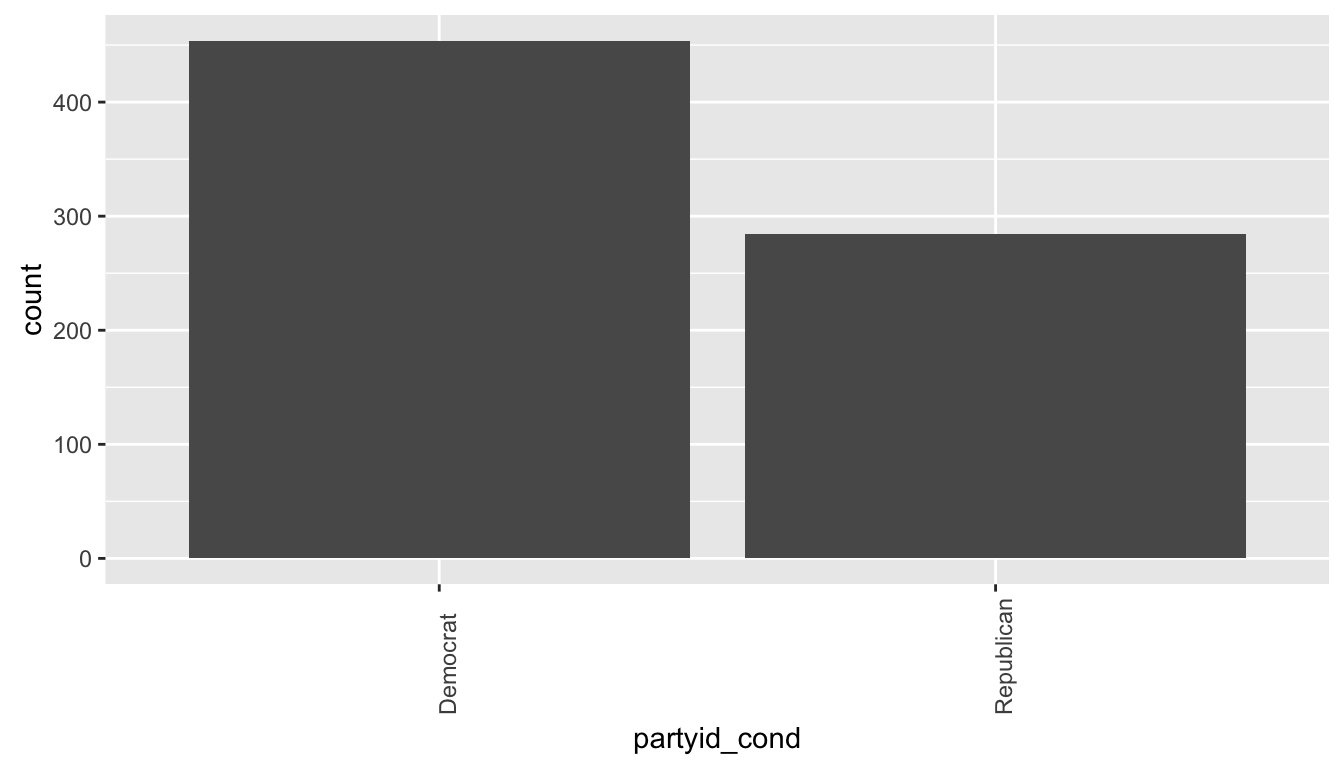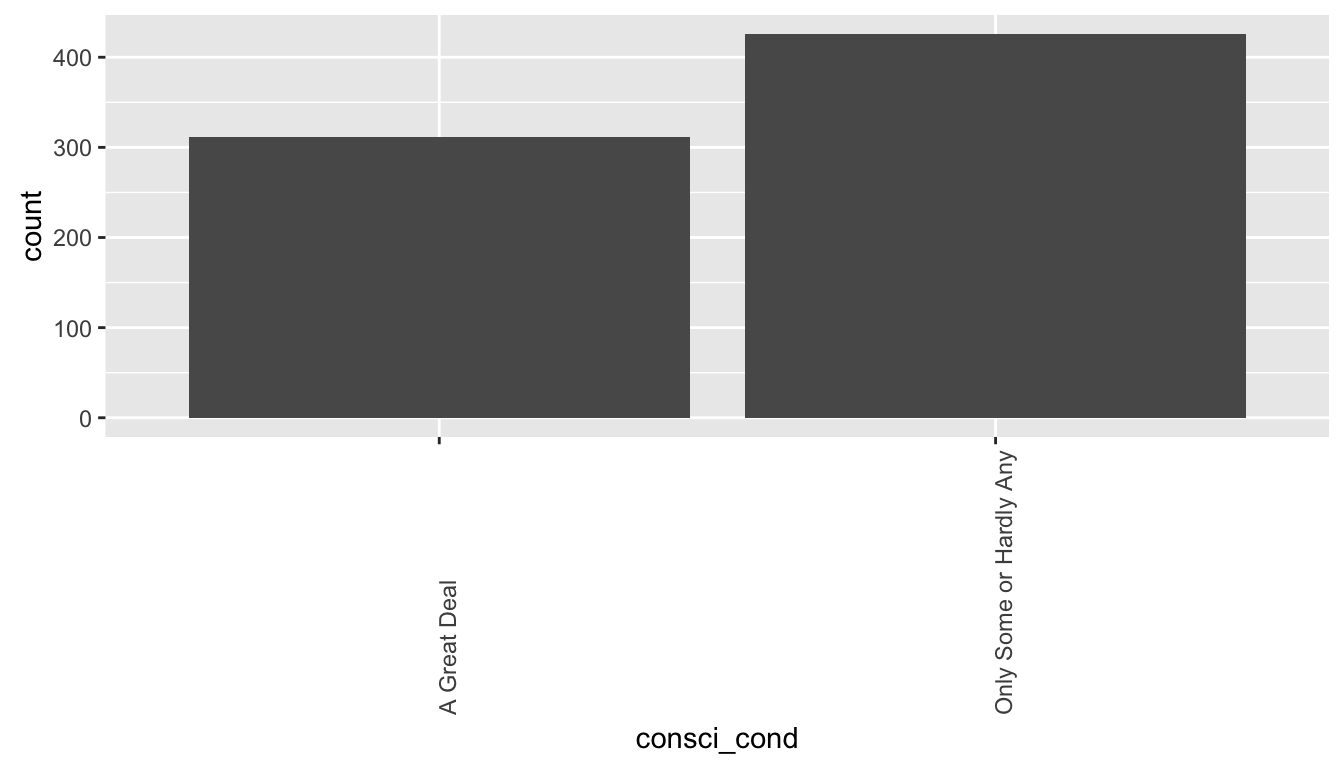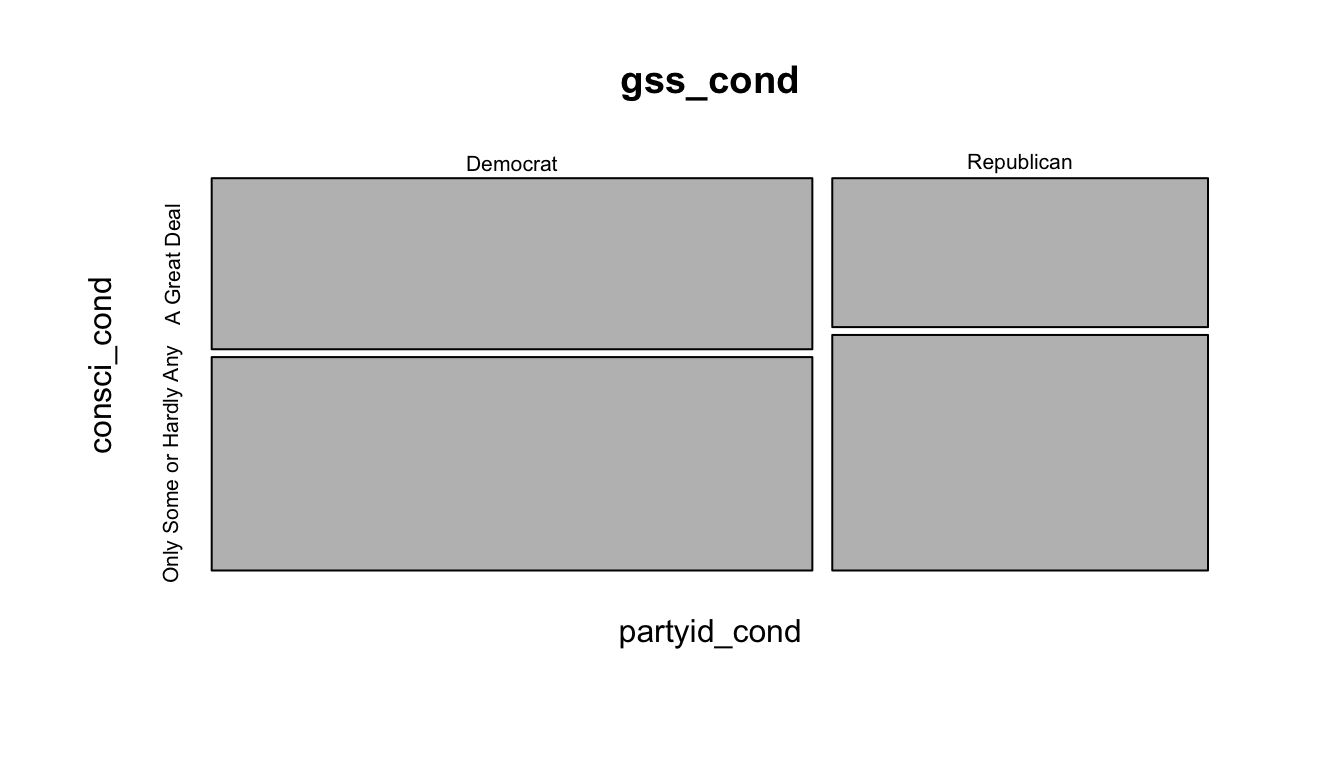## Setup

library(ggplot2)
library(dplyr)
library(statsr)

load("gss.Rdata")

## Assignment

Instructions

Your project will consist of 4 parts:

1. Data: (3 points) Describe how the observations in the sample are collected, and the implications of this data collection method on the scope of inference (generalizability / causality). Note that you might will need to look into documentation on the GSS to answer this question. See http://gss.norc.org/
2. Research question: (3 points) Come up with a research question that you want to answer using these data. You should phrase your research question in a way that matches up with the scope of inference your dataset allows for. You are welcomed to create new variables based on existing ones. Along with your research question include a brief discussion (1-2 sentences) as to why this question is of interest to you and/or your audience.
3. EDA: (10 points) Perform exploratory data analysis (EDA) that addresses the research question you outlined above. Your EDA should contain numerical summaries and visualizations. Each R output and plot should be accompanied by a brief interpretation.
4. Inference: (28 points) Perform inference that addresses the research question you outlined above. Each R output and plot should be accompanied by a brief interpretation.

In addition to these parts, there are also 6 points allocated to format, overall organization, and readability of your project. Total points add up to 50 points.

## Part 1: Data

The General Social Survey consists of observations from a random sample of the US population.

Random sampling allows for generalization to the US population. Causal inferences cannot be made because random assignment was not used for controlled experiments.

## Part 2: Research question

Is political party affiliation associated with confidence in the scientific community?

Some scientific issues become more prevalent in the public debate during presidential elections, like climate change or evolution and whether it should be taught in schools. An association or lack of association between these two variables could give us clues as to why they become key topics of debate.

Further, understanding why political groups have different views or opinions allows us to understand each other and work together more effectively as a society.

Variables used in this analysis: partyid, consci

partyid is political party affiliation with levels such as Democrat, Republican.
consci is the respondent’s level of confidence in the scientific community with levels such as A Great Deal of Confidence, Only Some, or Hardly Any.

## Part 3: Exploratory data analysis

### Data Cleaning

Subset the data to include only partyid and consci to create a condensed version of the dataset for this analysis.

Also, create condensed levels in the variables to eliminate granularity:

1. consci_cond
• A Great Deal (stays the same)
• Only Some or Hardly Any = Only Some + Hardly Any
1. partyid_cond
• Democrat = Str Democrat + Not Str Democrat
• Republican = Str Republican + Not Str Republican
• Independent = Ind,Near Dem + Independent + Ind,Near Rep
1. Remove observations where partyid == “Other Party” or “Independent”
2. Remove observations where partyid or consci is NA since we cannot analyze an association for these observations.
3. Filter on 2012 data, the most recent, to approximate current political views.
# Subset
gss_cond <- gss[c("partyid","consci","year")]

# New variables
gss_cond <- gss_cond %>%
mutate(partyid_cond = partyid, consci_cond = consci)

# Create condensed values for consci_cond
gss_cond$consci_cond <- as.character(gss_cond$consci_cond)
gss_cond$consci_cond[gss_cond$consci_cond == "Only Some"] <- "Only Some or Hardly Any"
gss_cond$consci_cond[gss_cond$consci_cond == "Hardly Any"] <- "Only Some or Hardly Any"
gss_cond$consci_cond <- as.factor(gss_cond$consci_cond)

# Create condensed values for partyid_cond
gss_cond$partyid_cond <- as.character(gss_cond$partyid_cond)
gss_cond$partyid_cond[gss_cond$partyid_cond == "Not Str Democrat"] <- "Democrat"
gss_cond$partyid_cond[gss_cond$partyid_cond == "Strong Democrat"] <- "Democrat"
gss_cond$partyid_cond[gss_cond$partyid_cond == "Ind,Near Dem"] <- "Independent"
gss_cond$partyid_cond[gss_cond$partyid_cond == "Ind,Near Rep"] <- "Independent"
gss_cond$partyid_cond[gss_cond$partyid_cond == "Ind,Near Rep"] <- "Independent"
gss_cond$partyid_cond[gss_cond$partyid_cond == "Not Str Republican"] <- "Republican"
gss_cond$partyid_cond[gss_cond$partyid_cond == "Strong Republican"] <- "Republican"
gss_cond$partyid_cond <- as.factor(gss_cond$partyid_cond)

# Remove "Other Party"
gss_cond$partyid_cond <- as.character(gss_cond$partyid_cond)
gss_cond <- gss_cond[!(gss_cond$partyid_cond == "Other Party"),] gss_cond <- gss_cond[!(gss_cond$partyid_cond == "Independent"),]
gss_cond$partyid_cond <- as.factor(gss_cond$partyid_cond)

# Remove rows with NA
gss_cond <- gss_cond[!(is.na(gss_cond$partyid_cond)),] gss_cond <- gss_cond[!(is.na(gss_cond$consci_cond)),]

# 2012 only
gss_cond <- gss_cond[(gss_cond\$year == 2012),]

### Political Party Affiliation

ggplot(gss_cond,aes(x=partyid_cond)) + geom_bar() + theme(axis.text.x=element_text(angle = 90, hjust = 0))The above bar plot shows the distribution of political party affiliation. “Democrat” has the highest frequency count. “Republican” has the lowest.

gss_cond %>%
group_by (partyid_cond) %>%
summarise (n=n()) %>%
mutate(rel.freq = paste0(round(100 * n/sum(n), 0), "%"))
## Source: local data frame [2 x 3]
##
##   partyid_cond     n rel.freq
##         (fctr) (int)    (chr)
## 1     Democrat   454      62%
## 2   Republican   284      38%

The frequencies and relative frequencies of each partyid. The number of respondents identifying as Democrat is 24 percentage points higher than then number identifying as Republican.

### Confidence in the Scientific Community

ggplot(gss_cond,aes(x=consci_cond)) + geom_bar() + theme(axis.text.x=element_text(angle = 90, hjust = 0))This bar plot shows the distribution of respondents’ confidence in the scientific community. “Only Some or Hardly Any” occurs more frequently than “A Great Deal”.

gss_cond %>%
group_by (consci_cond) %>%
summarise (n=n()) %>%
mutate(rel.freq = paste0(round(100 * n/sum(n), 0), "%"))
## Source: local data frame [2 x 3]
##
##               consci_cond     n rel.freq
##                    (fctr) (int)    (chr)
## 1            A Great Deal   312      42%
## 2 Only Some or Hardly Any   426      58%

The frequencies and relative frequencies of each partyid. 58% of respondents have only some or hardly any confidence in the scientific community. 16% fewer respondents have a great deal of confidence in the scientific community.

### Both variables together

gss_cond %>%
group_by (partyid_cond, consci_cond) %>%
summarise (n=n()) %>%
mutate(rel.freq = paste0(round(100 * n/sum(n), 0), "%"))
## Source: local data frame [4 x 4]
## Groups: partyid_cond 
##
##   partyid_cond             consci_cond     n rel.freq
##         (fctr)                  (fctr) (int)    (chr)
## 1     Democrat            A Great Deal   202      44%
## 2     Democrat Only Some or Hardly Any   252      56%
## 3   Republican            A Great Deal   110      39%
## 4   Republican Only Some or Hardly Any   174      61%

In the sample, 44% of respondents that are Democrats have a great deal of confidence in the scientific community. 39% of Republican respondents have a great deal of confidence in the scientific community.

mosaicplot(~partyid_cond + consci_cond, data = gss_cond)partyid is on the x-axis and consci is on the y-axis in this mosaic plot. It cannot be determined from this plot of sample data if there is an association in the population between party affiliation and confidence in the scientific community.

Statistical inference will be used to estimate the population parameter.

## Part 4: Inference

### Hypothesis

Is there an association between party affiliation and having a great deal of confidence in the scientific community?

We compare the proportion of democrats that have this view to the proportion of republicans. Since these are categorical variables with 2 levels we test the difference between proportions.

The first step is to calculate the difference between proportions, then compare that to the null distribution of the difference. We use the pooled proportion as our best estimate for obtaining the null value of a difference of 0.

We conduct a hypothesis test at a 5% significance level:

$$H_0:$$ The proportion of democracts in the population that have a great deal of confidence in the scientific community is equal to the number of repulicans that have a great deal of confidence in the scientific community.
$$H_A:$$ The population proportion is different among Democrats and Republicans when it comes to their confidence in the scientific community.

$$H_0: p_{Democrat} - p_{Republican} = 0$$
$$H_A: p_{Democrat} - p_{Republican} \neq 0$$

### Conditions

Independence: Success-Failure Condition We test that the proportions are equal but we don’t know what the proportions will be. In a case like this we use the pooled proportion to calculate the success-failure condition and obtain a null value to test the hypothesis.

\begin{aligned} \hat{p}_{pool} &= \frac{{successes}_{Democrat} + {successes}_{Republican}}{n_{Democrat} + n_{Republican}} \\\\ \hat{p}_{pool} &= \frac{202 + 110}{454 + 284} \\\\ \hat{p}_{pool} &= 0.423 \end{aligned}

Check the success failure condition:

$Democrats: 454 \times .423 = 192 \ge 10 \\ Democrats: 454 \times .577 = 262 \ge 10 \\ Republicans: 284 \times .423 = 120 \ge 10 \\ Republicans: 284 \times .577 = 164 \ge 10$

The success failure condition is met.

Independence: Within and between groups This condition is met because respondents were sampled randomly within groups and between groups. We have no reason to suspect sampled Democrats and Republicans are associated (dependent).

Independence: 10% condition 454 is less than 10% of all US Democrats and 284 is less than 10% of all US Republicans.

Normality The above conditions were met so we can assume the sampling distribution of the difference between two proportions is nearly normal.

### Methods

The normal distribution will be used to test the difference between proportions.

The test is used to find out if the population proportion of Democrats that have a great deal of confidence in the scientific community is equal to or different than the population proportion of Republicans that have a great deal of confidence in the scientific community.

Calculate the standard error:

\begin{aligned} \text{ } \\\\ SE_{(\hat{p}_d-\hat{p}_r)}&=\sqrt{\frac{\hat{p}_{pool}(1-\hat{p}_{pool})}{n_d}+\frac{\hat{p}_{pool}(1-\hat{p}_{pool})}{n_r}} \\\\ SE_{(\hat{p}_d-\hat{p}_r)}&=\sqrt{\frac{0.423\times(1-0.423)}{454}+\frac{0.423\times(1-0.423)}{284}} \\\\ SE_{(\hat{p}_d-\hat{p}_r)}&=0.0374 \end{aligned}

Calculate the point estimate:

\begin{aligned} \text{point estimate} &= \hat{p}_d - \hat{p}_r \\\\ \text{point estimate} &= 0.445 - 0.387 \\\\ \text{point estimate} &= 0.0576 \end{aligned}

Calculate a confidence interval. At 95% confidence, z* = 1.96:

\begin{aligned} CI &= 0.0576 \pm 1.96 \times 0.0374 \\\\ CI &= (-0.016, 0.131) \end{aligned}

We are 95% confident that the difference in the proportion is between -0.016, 0.131.

Calculate the test statistic:

\begin{aligned} Z &= \frac{0.0576 - 0}{0.0374} \\\\ Z &= 1.541 \end{aligned}

Calculate the p-value:

\begin{aligned} \text{p-value} &= P( |Z| > 1.541) \\\\ \text{p-value} &= 0.123 \end{aligned}

pnorm(1.541,lower.tail=FALSE)*2
##  0.1233168

(See Conclusions)

### Code

Alternatively, the R function prop.test will test the difference in proportions:

prop.test(c(202,110),c(454,284),alternative="two.sided")
##
##  2-sample test for equality of proportions with continuity
##  correction
##
## data:  c(202, 110) out of c(454, 284)
## X-squared = 2.1459, df = 1, p-value = 0.143
## alternative hypothesis: two.sided
## 95 percent confidence interval:
##  -0.01804972  0.13326967
## sample estimates:
##    prop 1    prop 2
## 0.4449339 0.3873239

The R code yields similar results. The p-value is about 14%. The confidence interval varies a bit due to rounding but it similarly includes 0.

### Conclusions

The probability is 12.3% (or 14.3% using prop.test) that the difference between the proportions is $$.0576$$ given the null hypothesis that the difference is 0. There is not sufficient evidence to reject the null hypothesis in favor of the alternative and the difference in the proportions could be due to sampling variability.

The proportion of Democrats in the population that have a great deal of confidence in the scientific community is estimated to be equal to the same proportion of Republicans.

This conclusion is consistent with the confidence interval of $$(-0.016, 0.131)$$ which includes 0.

This may be a clue as to why scientific issues are debated during election periods but knowing for certain why some issues are debated and others are not is a further area of research. Perhaps, for example, Democrats and Republicans have equal amounts in faith in contrasting studies and debate the results of those studies rather than their confidence in them generally.